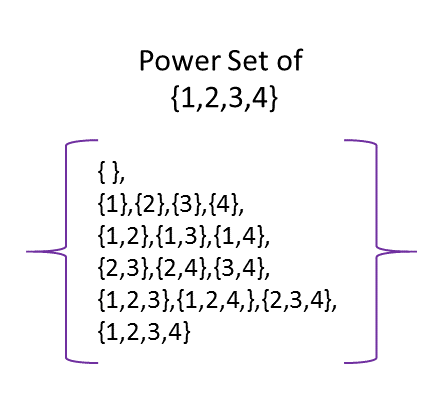# Definition of Power SetA power set is the set of all subsets of a set.

For example, the subsets of the set $S = \{1,2\}$ are:

• The empty set $\{\;\}$
• The one element sets $\{1\}$ and $\{2\}$
• And the whole set $\{1,2\}$
If we put them all together, we get the power set:
$P(S) = \{ \{\;\},\{1\},\{2\}, \{1,2\}\}$

### Description

The aim of this dictionary is to provide definitions to common mathematical terms. Students learn a new math skill every week at school, sometimes just before they start a new skill, if they want to look at what a specific term means, this is where this dictionary will become handy and a go-to guide for a student.

### Audience

Year 1 to Year 12 students

### Learning Objectives

Learn common math terms starting with letter P

Author: Subject Coach
You must be logged in as Student to ask a Question.